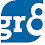# Mental Maths (Comparison of Fractions) - Part 2

### Model 5 :

• For a fraction Less than 1 :
• If the difference between the numerator and the denominator is same then the fraction with the larger values of numerator and denominator will be the largest. Have a look at the following example.
• For a fraction Greater than 1 :
• If the difference between the numerator and denominator is same, then the fraction with the smaller values will be the largest.
• Which of the following fraction is largest ?
• (31/27), (43/39), (57/53), (27/23), (29/25)
• As the difference between the numerator and the denominator is same, the fraction with the smaller values i.e., 27/23 is the largest.

### Which of the following fractions is the largest? (15/17), (23/29), (31/34), (11/15) Comparing fractions 15/17 and 23/29 The numerator of the fraction has increased from 15 to 23. i.e. 8/15 i.e. a little more than 50%.  The denominator of the fraction has increased from 17 to 29. i.e., 12/17 i.e., well over 50%. As the percentage increase in the numerator is less than the  percentage increase in the denominator, the fraction 15/17 > 23/29. Now compare 15/17 with 31/34 As the change in the numerator is more than double (15 to 31), and the change in the denominator is exactly double, the fraction 15/17 < 31/34. Now compare 11/15 and 31/34 The numerator has almost tripled from 11 to 31 whereas the denominator has just over doubled from 15 to 34. Since the increase in numerator is greater than the increase in the denominator, 31 /34 > 11/15. So, 31/34 is the largest fraction :) Thats all for now friends.... If you have any doubts about this post please feel free to use the comments section or mail us at guide4bankexams@gmail.com. If you feel this post interesting, feel free to share with your friends. Happy Reading :)

1.plz clearly explain abt the model 6 in comparison of fractions.

1.@ Priya, EDITED the post. hope you can clearly understand the process now. If no, then plz let us know, we'll try to explain the process with a new detailed example

Good Day

2.Superb Yaar . I always find it difficult to solve the fractions and try to solve by doing lenghty stupid calculation. but your all methods are superb.all are very much helpful.
thanks man.nice job

3.superb mam you are just awsome i did not attend this question by thinking that these are very tough but now after reading this just less than 1 minute i can get the answer u r awsome thanks a lot please also help me in english and general awareness section plzz
thanks a lot

1.sure friend. Keep visiting :)

4.mam point mai rakh ke nahi kar sakte hai kya??

1.Ofcourse you can Friend. Here the thing is to get the answer in Quick manner. You can use any method to get quick answers according to your convenience.

5.awesome mam

6.7.thank you so much mam it is very useful

8.thank you so much it is very useful by the same way can you publish or post the tricks for reasoning and English statements

1.Already posted Friend. Just check in the appropriate sections above.

9.thanku so much for helping us

10.some thing differ ... good

11.fantastic!!!...Thank You very useful site...:)

12.Thank you for making fractions easier:)

13.thanq very much

14.for Model 6 you may also try the butterfly technique...it will make the problems of this kind simpler than the one mentioned here.

15.super super fantastic minmblowing.......

16.How can i Know that the fraction is greater /less then 1??

17.18.19.20.